# Electronics and Communication Engineering - Exam Questions Papers

31.

The maximum effective aperture of an antenna which is operating at wavelength of 2 m and has a directivity of 100 is __________ .

 A. 15.92 B. 31.84 C. 3.184 D. 1.592

Explanation:

The maximum effective aperture is given by: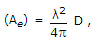D = 100, λ = 2 m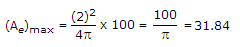.

32.

A speech signal, band limited to 4kHz and peak voltage varying between + 5V and - 5V is sampled at the Nyquist rate. Each sample is quantized and represented by 8 bits. Assuming the signal to be uniformly distributed between its peak values, the signal to noise ratio at the quantizer output is

 A. 156 dB B. 32 dB C. 48 dB D. 64 dB

Explanation:

SNR = 6n + 1.7 = 6 x 8 + 1.7 = 48 + 1.7 .

33.

The drain current of a MOSFET in saturation is given by lp = K(VGS - VT)2 where K is a constant. The magnitude of the transconductance gm is

 A.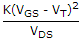B. 2K (VGS - VT) C.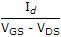D.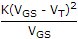Explanation:

ID = k(VGS - VT)2,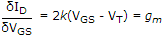.

34.

Two infinitely long wires carrying current are as shown in the figure below. One wire is in the y-z plane and parallel to the y-axis. The other wise is in the x-y plane and parallel to the x-axis. Which components of the resulting magnetic field are non-zero at the origin?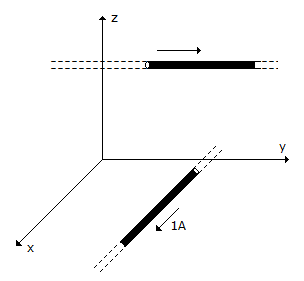A. x, y, z components B. x, y components C. y, z components D. x, z components

Explanation:

Apply right hand thumb rule.

35.

A uniform plane wave is described by the equation H =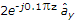A/m. If the velocity of the wave is 2 x 108 m/s and εr = 1.8, then. The electric field intensity is

 A. 90.35e-j0.1px v/m B. 903.5e-j0.1px v/m C. 903.5e-j0.1pz v/m D. 90.35e-j0.1pz v/m

Explanation:

Using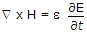, we have,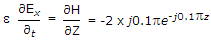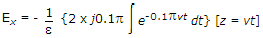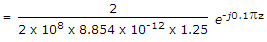= 903.5e-j0.1pzv/m.

#### Current Affairs 2022

Interview Questions and Answers